Ever tried to help your child with primary math homework and got stumped? Today’s math questions can be challenging – even for adults.

Math education is changing. While many parents spent time memorising procedures and formulas, today’s students are expected to not only understand and master the concepts but also to have strong thinking skills and problem-solving skills to solve complex math questions.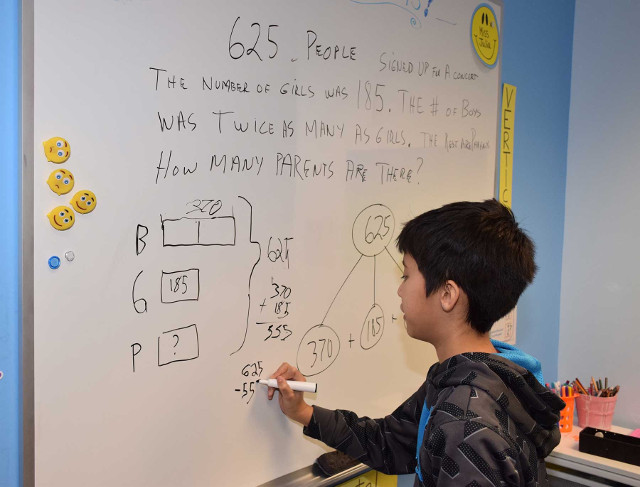As new concepts and strategies are being taught, and homework turns from arithmetic exercises to using multiple ways to solve a math word problem, you may feel unsure or have no idea how to help your child.

In this series, the curriculum team at Seriously Addictive Mathematics (S.A.M) shares expert tips on math heuristics and how to use them to solve math word problems.

What Are Heuristics?

Heuristics – a word that baffles many primary school students and their parents.

To define it simply, math heuristics are strategies that students can use to solve complex word problems.

Word problems can be solved in several ways using different heuristics, while some word problems are solved using a combination of heuristics.

To solve word problems efficiently, students must be familiar with both the problem-solving strategies (heuristics) and the problem-solving process.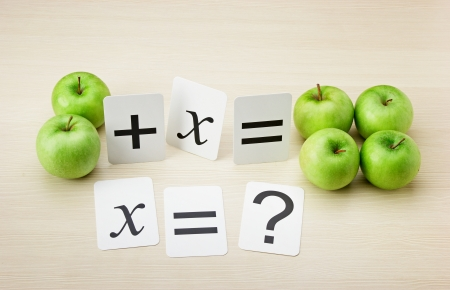How Many Heuristics Are There?

In Singapore Math, there are 12 heuristics in the primary math syllabus that can be grouped into four main categories:

• To give a representation: Draw a diagram/model, draw a table, make a systematic list
• To make a calculated guess: Look for pattern(s), guess and check, make suppositions
• To go through the process: Act it out, work backwards, use the before-after concept
• To change the problem: Restate the problem in another way, simplify the problem, solve part of the problem

What Is The Problem-Solving Process?Another important point to note is that Singapore Math adopts Polya’s four-step problem-solving process:

1. Understand the problem: What to find? What is known and unknown?
2. Devise a plan: Choose the most suitable heuristic
3. Carry out the plan: Solve the problem
4. Look back: Check the answer

So, how can we use heuristics to solve math word problems? Here are examples of word problems with solutions provided by the curriculum team at S.A.M.

Heuristic: Act it out

Word Problem (Primary 1):

Alan, Ben and Carol are in the school’s Art Club. Their teacher, Mr Tan, wants two of them to join a contest. How many ways can Mr Tan choose two pupils?

Solution:

Alan and Ben, Alan and Carol, Ben and Carol.

Mr Tan can choose two pupils in 3 ways.

Heuristic: Draw a diagram/model

Word Problem (Primary 3):

The smaller of the two numbers is 1217. The greater number is 859 more than the smaller number.
(a) What is the greater number?
(b) What is the sum of the numbers?

Solution: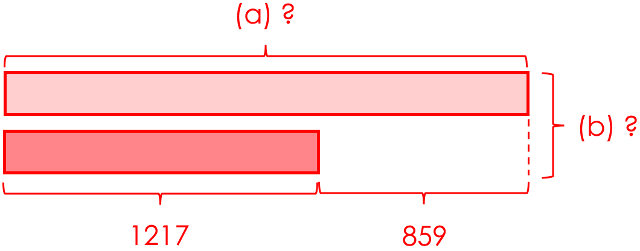The greater number is 1217 + 859 = 2076.

The sum of the numbers is 2076 + 1217 = 3293.

Heuristic: Look for pattern(s)

Word Problem (Primary 1):

Draw the shape that comes next.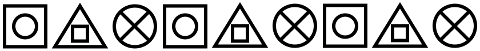Solution:

Label all shapes, the pattern is in repeating blocks of A, B, C.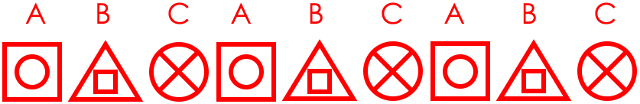The next shape isHeuristic: Make a systematic list

Word Problem (Primary 2):

A shop sells apples in bags of 3. It sells lemons in bags of 4. Paul buys some bags of apples and lemons. He buys the same number of each fruit. He buys more than 20 and fewer than 30 pieces of each fruit. How many apples does Paul buy?

Solution:

 Number of apples Number of lemons 1) 3                6) 18 1) 4                6) 24 2) 6                7) 21 2) 8                7) 28 3) 9                8) 24 3) 12 4) 12              9) 27 4) 16 5) 15              10) 30 5) 20

Heuristic: Guess and check

Word Problem (Primary 5):

Vijay is presented with the equations below. Insert one pair of brackets in each equation to make it true.
4 × 11 + 18 ÷ 3 + 6 = 46

Solution:

 Way Answer Check 1 4 × (11 + 18) ÷ 3 + 6         = 4 × 29 ÷ 3 + 6                                              = 116 ÷ 3 + 6 116 is not divisible 3. ✗ 2 4 × 11 + 18 ÷ (3 + 6)          = 4 × 11 + 18 ÷ 9                                               = 44 + 18 ÷ 9                                               = 44 + 2                                               = 46 ✓

The equation is 4 x 11 + 18 ÷ (3 + 6) = 46.

Heuristic: Restate the problem in another way

Word Problem (Primary 2):

There are some identical pens and erasers. 2 pens and 3 erasers are 45 centimetres long altogether. 6 erasers and 2 pens are 60 centimetres long altogether. What is the length of 3 erasers?

Solution:

If we subtract the total length of 2 pens and 6 erasers from that of 2 pens and 3 erasers, we get the length of 3 erasers.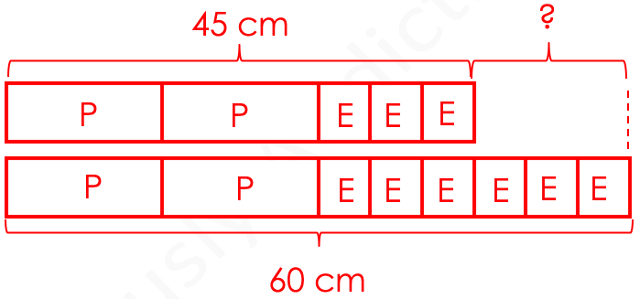60 cm – 45 cm = 15 cm

The length of 3 erasers is 15 cm.

Heuristic: Solve part of the problem

Word Problem (Primary 6):

The diagram below shows 4 shaded triangles in Triangle ABC. All the triangles in the diagram are equilateral triangles. If the area of Triangle ABC is 64 cm2, find the total area of the shaded triangles.Solution: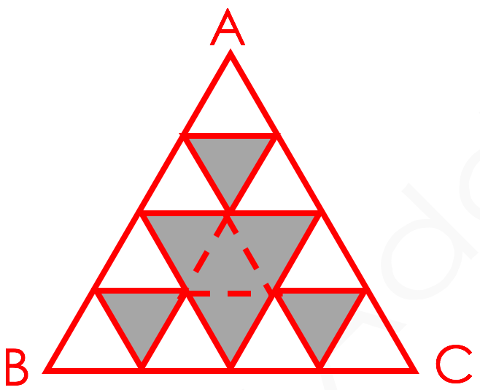Triangle ABC is made up of 16 small equilateral triangles.
7 out of 16 small equilateral triangles are shaded.
716 × 64 = 28

The total area of the shaded triangles is 28 cm2.

Heuristic: Simplify the problem

Word Problem (Primary 6):

The shaded figure below shows a semicircle and two-quarter circles. Find the area of the shaded figure. (Take pi = 3.14)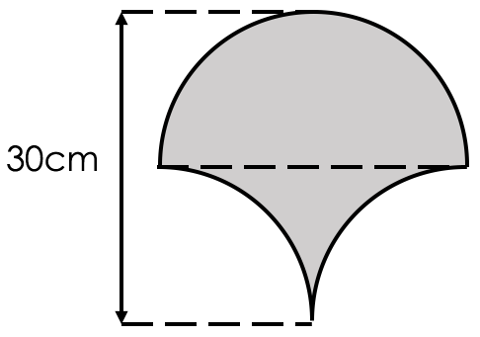Solution: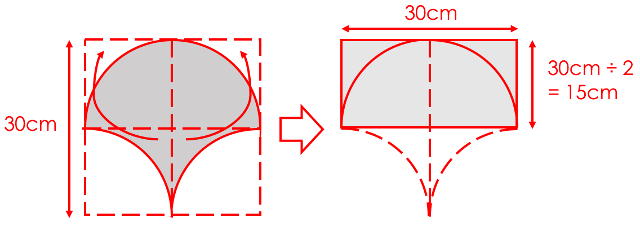30 × 15 = 450

The area of the shaded figure is 450 cm2.

Heuristic: Work backwards

Word Problem (Primary 3):

Darren had some stickers in his collection. He bought 20 more stickers and gave 33 stickers to his sister. He had 46 stickers left. How many stickers did Darren have in his collection at first?

Solution:

Label the changes as C1 and C2.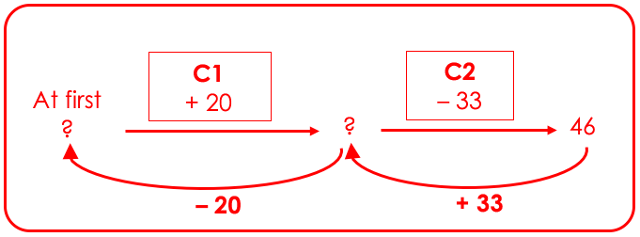Before C2: 46 + 33 = 79
At first: 79 – 20 = 59

Darren has 59 stickers in his collection at first.

Heuristic: Draw a table

Word Problem (Primary 4):

Janice wanted to distribute stickers equally among some children. If each child received 8 stickers, she would have 3 stickers left. If each child received 11 stickers, she would need another 9 stickers. How many children were there?

Solution:

 Number of children 1 2 3 4 … Multiples of 8 8 16 24 32 … Multiples of 11 11 22 33 44 … Multiples of 8 (+ 3) 11 19 27 35 … Multiples of 11 (- 9) 2 13 24 35 …

Janice had 35 stickers. There were 4 children.

Heuristic: Make suppositions

Word Problem (Primary 4):

Farmer James has some ducks, horses and cows on his farm. He has 30 ducks and cows altogether. The total number of legs the ducks and cows have is 82. The total number of legs the horses have is 28. How many ducks and how many cows are there on the farm?

Solution:

Suppose that James has 30 ducks.
30 × 2 = 60
30 ducks have 60 legs altogether.

82 – 60 = 22
The total number of legs is 22 less than the actual total number.
A cow has 2 more legs than a duck.
22 ÷ 2 = 11
James has 11 cows.

30 – 11 = 19

There are 19 ducks and 11 cows on the farm.

Heuristic: Use before-after concept

Word Problem (Primary 5):

Ray and Sam each brought some money for shopping. The ratio of the amount of Ray’s money to the amount of Sam’s money was 3 : 4. After each of them bought a laptop for \$1250, the ratio of the amount of Ray’s money to the amount of Sam’s money became 1 : 3. How much money did Sam bring for shopping?

Solution: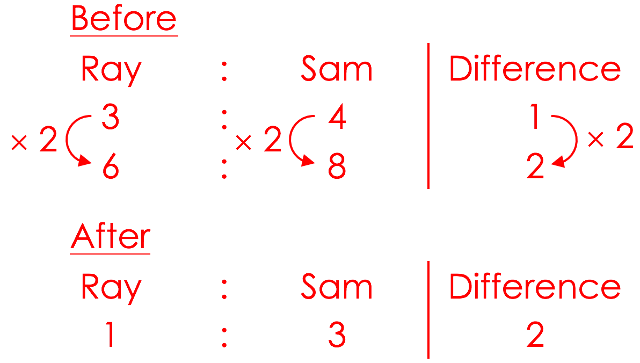(8 – 3) = 5 units = \$1250
8 units = \$1250 ÷ 5 × 8 = \$250 × 8 = \$2000

Sam brought \$2000 for shopping.

This is the first part to S.A.M Heuristics series for expert tips on math heuristics.

Read the rest of the “S.A.M Math Heuristics” five-part series below:

Established in 2010, Seriously Addictive Mathematics (S.A.M) is the world’s largest Singapore Math enrichment program for children aged four to 12. The award-winning S.A.M program is based on the global top-ranking Singapore Math curriculum with a focus on developing problem-solving and thinking skills.

The curriculum is complemented by S.A.M’s two-pillared approach of Classroom Engagement and Worksheet Reinforcement, with an individual learning plan tailored to each child at their own skill level and pace, because no two children learn alike.

This post is brought to you by Seriously Addictive Mathematics.

* * * * *

Like what you see here? Get parenting tips and stories straight to your inbox! Join our mailing list here.

Want to be heard 👂 and seen 👀 by over 100,000 parents in Singapore? We can help! Leave your contact here and we’ll be in touch.